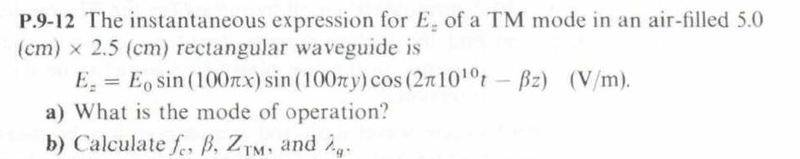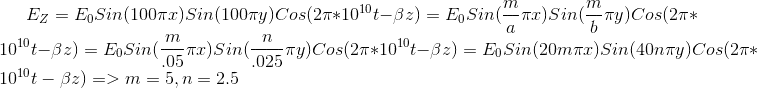# Find TM waves mode in a rectangular waveguide

## Homework Statement## Homework Equations[/B]

## The Attempt at a Solution

[/B]
Hello
As you see my solution I found TM(5 , 2.5) that is incorrect because n should be integer not float number.In Addition when I check book solution I found TM mode is TM(2,1)
what is my mistake?

Have you checked the errata for the edition of the book you are using. Seems to me there is a typo in their problem statement (which doesn't even tell you what units to use for the coordinates $x$ and $y$. What textbook is this from?